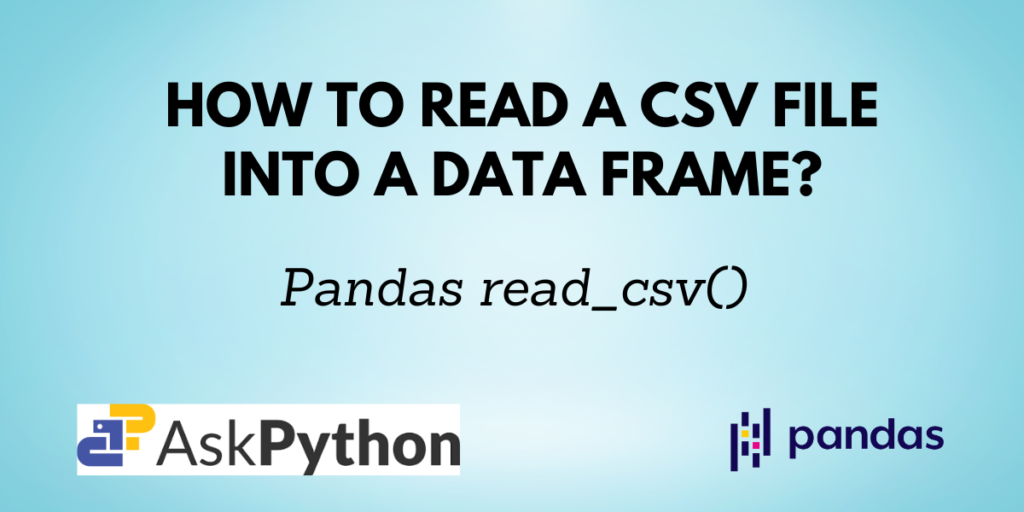In today’s world, data is available in abundance. Often we find it in the tabular format of CSV files. CSV files are nothing but Comma Separated Values files. You can convert these Comma Separated Values files into a Pandas DataFrame object with the help of the `pandas.read_csv()` function.

In this article, you will learn how to use the Pandas `read_csv` function and its various parameters using which you can get your desired output.

```pd.read_csv(filepath_or_buffer, sep=’ ,’ , header=’infer’,  index_col=None, usecols=None, engine=None, skiprows=None, nrows=None)
```

Returns:

A DataFrame with labeled axes.

Note: Please refer to the link in the ‘Reference’ section for the complete parameter list.

## Reading a CSV File without Parameters

Let us first see the sample CSV file named ‘data.csv’.

To read this file using Python, use the below function:

```import pandas as pd

df
```

Output:

Note that, if the CSV file you want to read is not in the same directory as your code file, you need to specify its file path instead of just the name of the file.

## Reading CSV files with Custom Separator

Instead of using a comma as a separator, you can use any other symbol as well to separate values in a CSV file. In such a case, you can specify the separator using the sep parameter.

Suppose you take a few entries from the above sample CSV files and modify in the below format and save them as ‘data2.csv’:

```fruit/colour/quantity/price
apple/red/2/120
banana/yellow/1/40
cherry/red/1/23
orange/orange/5/65
```

You can read this file as:

```df = pd.read_csv('data2.csv', sep = '/')

df
```

Output:

## Displaying only some specific columns in the output

If the dataset is too huge and you don’t want all the columns, you can select the columns that you need using the usecols parameter.

```df = pd.read_csv('data.csv', usecols=['fruit', 'quantity'])

df
```

Output:

As the code suggests, only the columns ‘fruits’ and ‘quantity’ are displayed and other columns are not included in the resulting DataFrame.

## Reading only ‘n’ rows of a CSV file using Pandas

Again if the dataset is too big and you don’t want all the records, you can assign an integer value to the nrows parameter, which then makes sure that only the first n rows are included in the output DataFrame.

In the below code, since n=8, only the first 8 rows are included in the output.

```df = pd.read_csv('data.csv', nrows = 8)

df
```

Output:

## Skipping rows while reading CSV

You can also skip some rows from the CSV file by using skiprows parameter. It can take an integer or a list of integers which represents the row numbers that are to be skipped.

```df = pd.read_csv('data.csv', skiprows = [4, 5, 11])

df
```

Output:

The rows grapes (index=3), watermelon (index=4) and guava (index=10) are skipped and not included in the result.

## Set a column as the index of the DataFrame

The index_col function lets you specify the column from the CSV file which you want as the index column of the DataFrame.

```df = pd.read_csv('data.csv', index_col = 'fruit')

df
```

Output:

If you observe the output, you will notice that instead of the default index as 0, 1, 2, …, n-1 the index here is the fruit columns from the CSV file.

## Conclusion

The `pandas.read_csv()` function lets you read any Comma Separated File (CSV) into a Pandas DataFrame object. It also provides various parameters which you can use to customize the output as per your requirements, some of which were discussed in this tutorial.